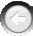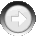﻿ SetEnumOperators

# SetEnumOperators

Navigation:  »No topics above this level«

# SetEnumOperatorsSetEnumOperators(Population As Integer, CrossoverType As Integer, EnumCrossoverRate As Single, EnumMutationRate As Single)   Purpose: This function sets the parameters of the basic genetic algorithm operators required for enumerated chromosomes.   Arguments: Population is the number of the population for which you are going to set the parameters of the operators. EnumCrossoverType is the number, either 0 or 1, which specifies the type of crossover for enumerated chromosomes.  Type 0 corresponds to the simple crossover with a single crossing point.  Type 1 corresponds to the so called 'Partially Matched Crossover' with 2 unequal crossing points.  Type 1 crossover is used for enumerated chromosomes of type 1 (unique representation) only.  You may use the symbolic constants REPEATING_GENES_CROSS and UNIQUE_GENES_CROSS for these values defined in the module GALIB32.BAS. EnumCrossoverRate is the rate of crossover, i.e., the probability that the crossover operator will be applied to an enumerated chromosome.  The probability is calculated per enumerated chromosome.  If an individual in the population has a number of enumerated chromosomes, the probability of crossover per individual is given by the expression: total crossover rate = 1-(1-enum crossover rate)^n,   where total crossover rate is the probability of crossover per individual, enum crossover rate is the probability of crossover per enumerated chromosome, and n is the number of enumerated chromosomes per individual.  Usually, values in the range 0.8-1.0 are appropriate. EnumMutationRate is the rate of mutation per element in an enumerated chromosome.  Enumerated chromosomes can have different lengths (number of integer elements), so the probability of mutation per chromosome is given by the expression:   total_mutation_rate = 1 - (1-enum_mutation_rate)^k,   where total_mutation_rate is the probability of chromosome mutation, enum_mutation_rate is the rate of mutation per chromosome element, and k is the length of a chromosome.  The probability of mutation of an individual is calculated by:   total_mutation_rate = 1-(1- enum_mutation_rate)^k,   but k in this case stands for the total length of all enumerated chromosomes of an individual.  Usually values 0.001-0.01 are appropriate.   Example: i = SetEnumOperators(PopNum, UNIQUE_GENES_CROSS, 0.9, 0.005).   This example sets the parameters of the genetic algorithm operators for the population PopNum.  New offspring will be generated by partially matched crossover.  The crossover rate will be 0.9, and the mutation rate will be 0.005.   Related Functions: SetOperators, SetStrategy